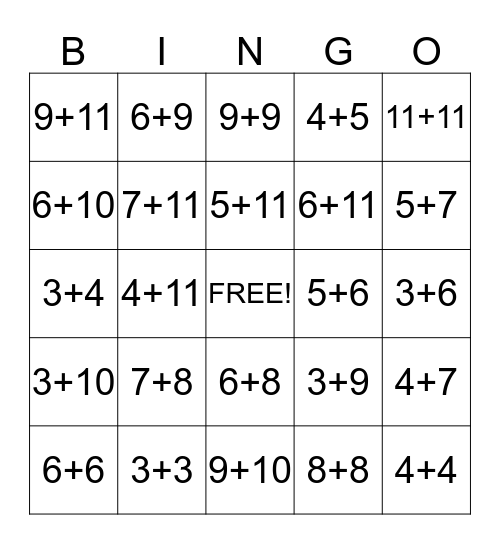# Hickman's Addition BingoThis bingo card has a free space and 45 words: 3+2, 4+4, 5+5, 6+6, 7+7, 8+8, 9+9, 10+10, 11+11, 3+3, 4+5, 5+6, 6+8, 7+8, 8+9, 9+10, 10+11, 3+4, 4+6, 5+7, 6+9, 7+9, 8+10, 9+11, 3+5, 3+6, 3+7, 3+8, 3+9, 3+10, 3+11, 4+7, 4+8, 4+9, 4+10, 4+11, 5+8, 5+9, 5+10, 5+11, 6+10, 6+11, 7+10, 7+11 and 8+11.

## Play Online